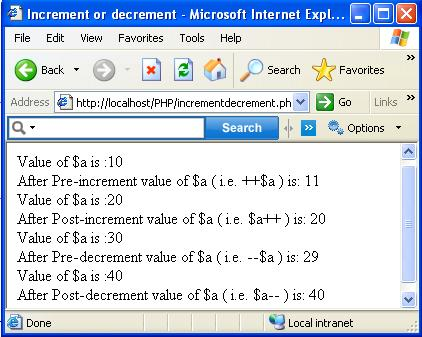PHP Programing language

Incrementing And Decrementing Examples
 Previous Home Next

In PHP is to increment (add 1 to) or decrement (Subtract 1 from ) values. Mainly there are more type to write a syntax for incrementing and decrementing like as:

```
<?php
\$a=1;
\$a=\$a+1;
?>
//If you using shortcut assignment operator += like as:
<?php
\$a=1;
\$a += 1;
?>
//And another way using for incrementing values:
the ++ operator, which you use like this:
<?php
\$a =1;
\$a++;
?>
```

Similarly this process is same way to work for decrement.

Note:If you using the ++ or -- operator after a variable, then the value in the variable is incremented after the rest of the statement is executed. If you put ++ or -- in front of a variable, the value in that variable is incremented or decremented before the rest of the statement is executed.

 Operator Name Effect ++\$a Pre-Increment Increments \$a by 1, then returns \$a. \$a++ Post-Increment Returns \$a, then increments \$a by 1. --\$a Pre-Decrement Decrements \$a by 1, then returns \$a. \$a-- Post-Decrement Returns \$a, then decrements \$a by 1.

Example for Increment and Decrement
```
<html>
<title>Increment or decrement</title>
<body>
<?php
\$a = 10;
echo 'Value of \$a is :'.\$a;
echo '<br />After Pre-increment value of \$a ( i.e. ++\$a ) is: '.++\$a;
\$a = 20;
echo '<br />Value of \$a is :'.\$a;
echo '<br />After Post-increment value of \$a ( i.e. \$a++ ) is: '.\$a++;
\$a = 30;
echo '<br />Value of \$a is :'.\$a;
echo '<br />After Pre-decrement value of \$a ( i.e. --\$a ) is: '.--\$a;
\$a = 40;
echo '<br />Value of \$a is :'.\$a;
echo '<br />After Post-decrement value of \$a ( i.e. \$a-- ) is: '.\$a--;
?>
</body>
</html>
```
outputPrevious Home Next